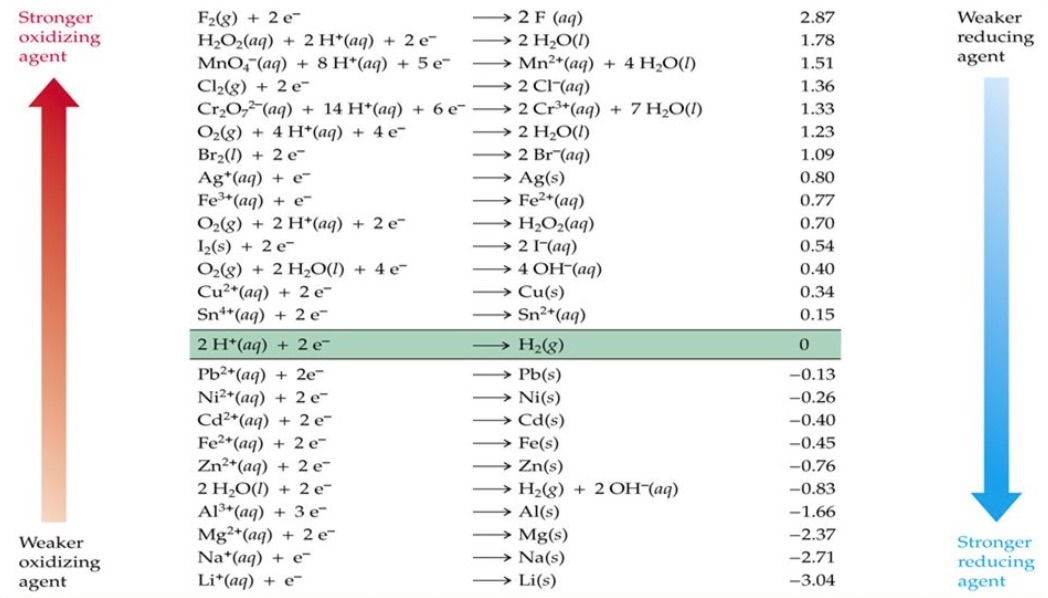# The Physics Behind Corrosion

In metal refining, energy is added to the metal ore to form a reduction reaction at the site and reduce the metal to a pure state. This is how we go from what looks like “rocks” to pure metal. In a corrosion reaction, the energy added during processing is released, and the metal returns to its oxide state. The added electrolytic properties of the salt in a marine environment make the reaction electrochemical in nature, shifting the speed and aggressiveness. This will be demonstrated through the comparison between the seawater vs. freshwater experiment.
In an oxidation reaction, the element loses an electron and becomes positively charged. In a reduction reaction, the element gains an electron and becomes negatively charged. These redox reactions come together to create a full electrochemical cell.

In an electrode, the anode is the site of oxidation, and the cathode is the site of reduction. The known cell voltages help us understand the movement of the electrons, as Ecell measures the potential of the reducing agent being oxidized and the oxidizing agent getting reduced.

E = E°ox + E°red

Using Gibb’s free energy equation, if E in this case is positive, then ΔG will be negative, meaning the reaction is possible. We add together the standard cell potentials for each of the components.In the experiment of steel in freshwater, we will have the half-cell reactions for iron and neutral water:

O2 + 2H2O +4e— = 4OH— where E° = +0.40
Fe2+ + 2e— = Fe where E° = -0.45

If we are testing for the corrosion of steel, we need to make the iron reaction the anode. We flip the direction of the reaction, as we want iron to be oxidized, so that

ox = +0.45
red = +0.40

Then, using

E = E°ox + E°E°red
= (0.45)+(0.40) = +0.85 V

Since E is positive, ΔG is negative, and the corrosion of steel is possible in this case. Given a table of standard half-cell reactions and potentials, students can complete this exercise for the examples in the demonstration, as well as for other examples we think of as a class. This is a basic rundown of the physics principles that will be covered in my lesson, along with exercises that students can work through problems on their own.